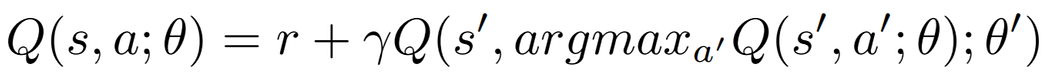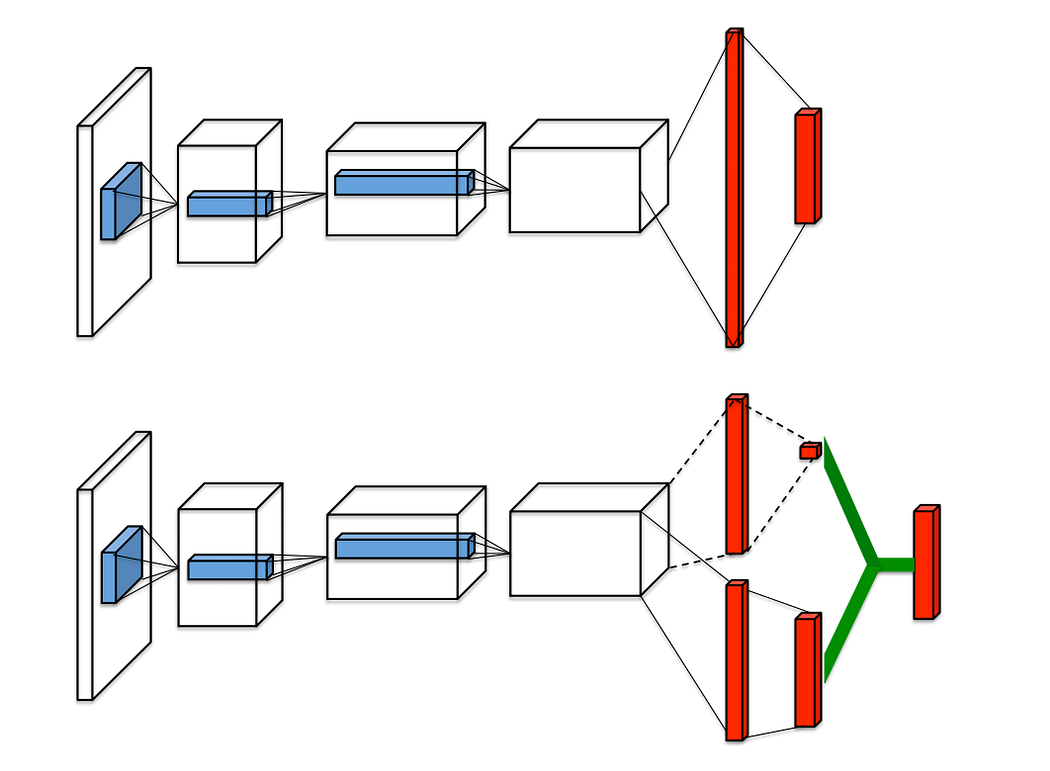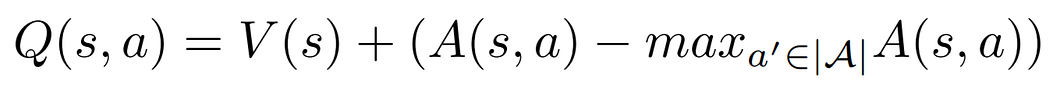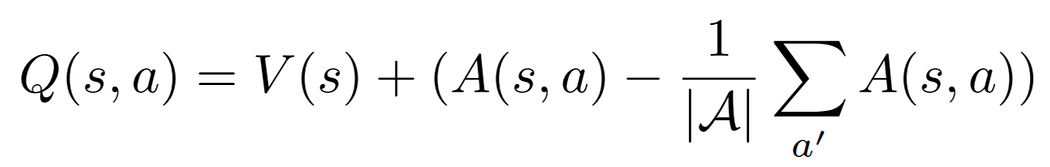2021-09-09
###### Best storage formats to save Pandas dataframes
2021-09-12In this part, we will see two algorithms that improve upon DQN. These are named Double DQN and Dueling DQN. But first, let’s introduce some terms we have ignored so far.

All the reinforcement learning (RL) algorithms can be classified into several families. The first one depends on whether the algorithm explicitly learns and/or uses the environment dynamics. If the algorithm uses these dynamics (also known as the model of the environment) during the decision-making process, then it will be a model-based algorithm. If it doesn’t make use of them, it will be model free. A model-based algorithm needs to learn (or be provided with) all the transition probabilities, which describe the probability of the agent transitioning from one state to another. As many environments are stochastic and their dynamics are unknown, the agent must learn the model behind it. Once the transition probabilities have been learned, it will use these probabilities to make better decisions. For instance, let’s say that from a given state with two available actions (a1 and a2), the probability of taking action a1 has a 0.9 probability of taking you to state A and you will get a reward of -10 there, and a 0.1 chance of taking you to state B and you will get a reward of +10; but action a2 has a probability of 1.0 of taking you to state C and you will obtain a reward of +5. In this situation, the best decision is to take action a2. Despite the reward in state B is larger, the probability of transitioning to that state is very low. Thus, not only is it important to take the rewards into account but the model as well. We will see more about model-based algorithms in the future.

The second family to classify these algorithms is whether they are off-policy or on-policy. The off-policy algorithms learn a value function independently from the agent’s decisions. This means that the behavior policy and the target policy can be different. The first one is the policy used by the agent to explore the environment and collect data, while the second one is the policy the agent is trying to learn and improve. This means that the agent can explore the environment completely randomly using some behavior policy, and that data can be used to train a target policy that can get a very high return. On the other hand, if we use an on-policy algorithm the behavior and target policies must be the same. These algorithms learn from the data that was collected by the same policy that we are trying to learn.

From now on, we will classify the algorithms in these families. For example, both the Q-Learning and DQN algorithms are model-free and off-policy. The two algorithms that we will see in this part, Double DQN and Dueling DQN, are also model-free and off-policy.

## Double DQN 

One of the problems of the DQN algorithm is that it overestimates the true rewards; the Q-values think the agent is going to obtain a higher return than what it will obtain in reality. To fix this, the authors of the Double DQN algorithm suggest using a simple trick: decoupling the action selection from the action evaluation. Instead of using the same Bellman equation as in the DQN algorithm, they change it like this:Image 1: Bellman equation for the Double DQN algorithm.

First, the main neural network θ decides which one is the best next action a’ among all the available next actions, and then the target neural network evaluates this action to know its Q-value. This simple trick has been shown to reduce overestimations, which results in better final policies.

## Dueling DQN 

This algorithm splits the Q-values in two different parts, the value function V(s) and the advantage function A(s, a).

The value function V(s) tells us how much reward we will collect from state s. And the advantage function A(s, a) tells us how much better one action is compared to the other actions. Combining the value V and the advantage A for each action, we can get the Q-values:

What the Dueling DQN algorithm  proposes is that the same neural network splits its last layer into two parts, one of them to estimate the state value function for state s (V(s)) and the other one to estimate the advantage function for each action a (A(s, a)), and at the end, it combines both parts into a single output, which will estimate the Q-values. This change is helpful because sometimes it is unnecessary to know the exact value of each action, so just learning the state-value function can be enough in some cases.Image 3. Image extracted from the Dueling DQN paper. Top: Regular DQN architecture. Bottom: Dueling DQN architecture.

However, training the neural network by simply summing the value and advantage functions is not possible. In Q=V+A, given the function Q, we cannot determine the values of V and A, since that is “unidentifiable”. To see a similar example, if I tell you that the value for Q is 20, and you need to know which two values sum up to 20 (20 = V + A), there are infinite possible solutions. To solve this, the paper suggests a trick: force the highest Q-value to be equal to the value V, thus making the highest value in the advantage function be zero and all other values negative. This will tell us exactly the value for V, and we can calculate all the advantages from there, solving the problem. This is how we would train it:Image 4: Trick to make the problem identifiable.

However, the paper suggests a small change to this procedure. Instead of calculating the max, it suggests that we replace it with the mean, so that’s what we will do (read the paper for more details). This is how we will train our network:Image 5: Alternative way to make the problem identifiable.

## The code

You can see the entire TensorFlow code and run it in this link (or the PyTorch version in this link).

We will solve one of the Atari 2600 games using OpenAI Gym. I have selected the game of Pong as it is a simple game to visualize and it is one of the easiest games to solve with DRL. We will use the code in this article as a reference. This time, we will change the architecture of the neural networks to split the last layer in two, using the value and advantage functions (named V and A in the code), and then combine them into the Q-values. We will also change the type of layers for the first few layers of the network. As we are learning directly from the pixels, a dense (or fully connected) neural network is not the best solution. We will use convolutional layers instead. The number of units and parameters of these layers will follow the same architecture as in the Dueling DQN paper:

```class DuelingDQN(tf.keras.Model):
"""Convolutional neural network for the Atari games."""
def __init__(self, num_actions):
super(DuelingDQN, self).__init__()
self.conv1 = tf.keras.layers.Conv2D(
filters=32, kernel_size=8, strides=4, activation="relu",
)
self.conv2 = tf.keras.layers.Conv2D(
filters=64, kernel_size=4, strides=2, activation="relu",
)
self.conv3 = tf.keras.layers.Conv2D(
filters=64, kernel_size=3, strides=1, activation="relu",
)
self.flatten = tf.keras.layers.Flatten()
self.dense1 = tf.keras.layers.Dense(units=512, activation="relu")
self.V = tf.keras.layers.Dense(1)
self.A = tf.kears.layers.Dense(num_actions)

@tf.function
def call(self, states):
"""Forward pass of the neural network with some inputs."""
x = self.conv1(states)
x = self.conv2(x)
x = self.conv3(x)
x = self.flatten(x)
x = self.dense1(x)
V = self.V(x)
A = self.A(x)
Q = V + tf.subtract(A, tf.reduce_mean(A, axis=1, keepdims=True))
return Q
```

Next, we will also change the function that performs the gradient descent step. We will modify it to implement the Double DQN training step instead of the regular DQN step. This means that the Bellman equation will be the one described above, which is slightly different from the original one.

```@tf.function
def train_step(states, actions, rewards, next_states, dones):
"""Perform a training iteration on a batch of data."""
# Select best next action using main_nn.
next_qs_main = main_nn(next_states)
next_qs_argmax = tf.argmax(next_qs_main, axis=-1)
next_action_mask = tf.one_hot(next_qs_argmax, num_actions)

# Evaluate that best action using target_nn to know its Q-value.
next_qs_target = target_nn(next_states)

# Create target using the reward and the discounted next Q-value.
target = rewards + (1. - dones) * discount * masked_next_qs
with tf.GradientTape() as tape:
# Q-values for the current state.
qs = main_nn(states)
action_mask = tf.one_hot(actions, num_actions)
loss = loss_fn(target, masked_qs)

return loss
```

We will run the main training loop in a similar manner to the previous parts, collecting data and saving it into the buffer to sample it later on. After training the network for 1000 episodes, this will be the result that gets printed. The return indicates how many goals each player has scored.

```Episode: 0/1000, Epsilon: 0.999, Loss: 0.0296, Return: -21.00
Episode: 100/1000, Epsilon: 0.897, Loss: 0.0008, Return: -20.21
Episode: 200/1000, Epsilon: 0.787, Loss: 0.0031, Return: -19.93
Episode: 300/1000, Epsilon: 0.655, Loss: 0.0025, Return: -18.96
Episode: 400/1000, Epsilon: 0.496, Loss: 0.0031, Return: -17.66
Episode: 500/1000, Epsilon: 0.297, Loss: 0.0026, Return: -15.86
Episode: 600/1000, Epsilon: 0.010, Loss: 0.0088, Return: -9.10
Episode: 700/1000, Epsilon: 0.010, Loss: 0.0057, Return: 5.80
Episode: 800/1000, Epsilon: 0.010, Loss: 0.0011, Return: 12.86
Episode: 900/1000, Epsilon: 0.010, Loss: 0.0008, Return: 15.87
Episode: 1000/1000, Epsilon: 0.010, Loss: 0.0011, Return: 18.98
```

And if we visualize the result by playing one more episode and recording the pixels, this will be how the agent behaves (our agent is the green one).

To see all the TensorFlow code and run it yourself, follow this link (or this link for the PyTorch version).

## References:

https://markelsanz14.medium.com/introduction-to-reinforcement-learning-part-4-double-dqn-and-dueling-dqn-b349c9a61ea1

https://towardsdatascience.com/dueling-deep-q-networks-81ffab672751

https://medium.com/@parsa_h_m/deep-reinforcement-learning-dqn-double-dqn-dueling-dqn-noisy-dqn-and-dqn-with-prioritized-551f621a9823##### Amir Masoud Sefidian
Machine Learning Engineer